Congruence
Triangle Proofs
Segment Proofs
Angle Proofs
100

If

\triangleCFE\cong\trianglePTR, \overline{CE}\cong?

\overline{PR}?

100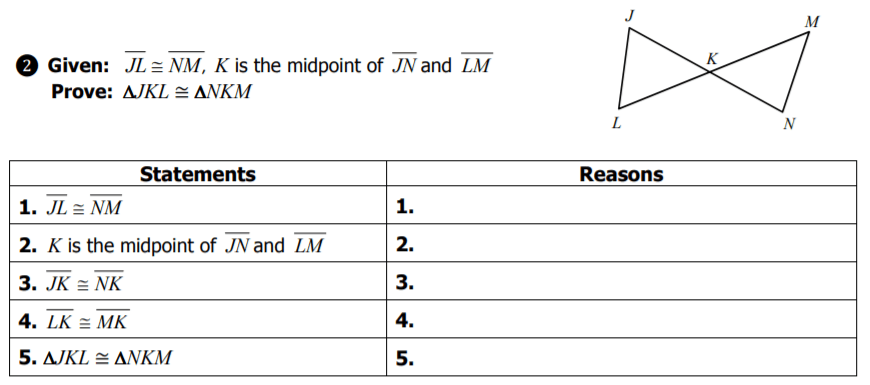Reasons 1 and 2

Given

100

Reasons 1 and 2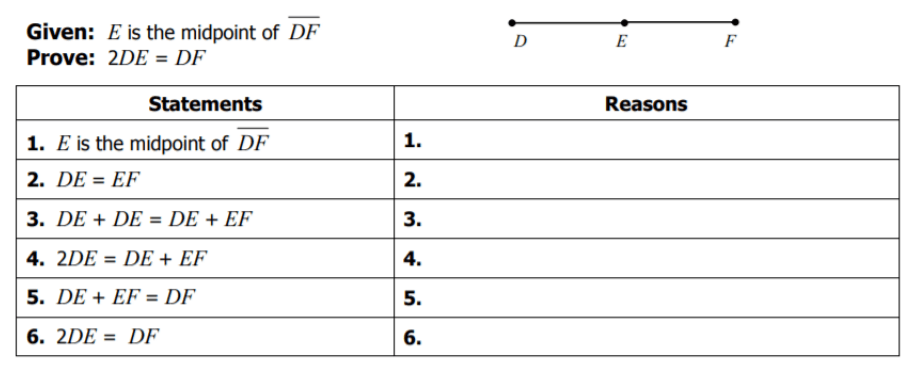Given and definition of midpoint

100

Reasons 1 and 2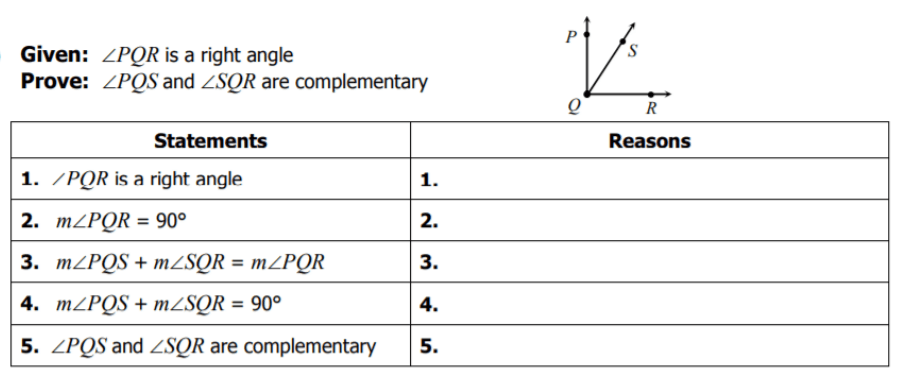Given and definition of right angle

100

A type of quadrilateral that is NOT a parallelogram.

(e.g. Kite, trapezoid)

200Triangle congruence postulate that proves

\trianglePQR\cong \trianglePQS

SSS

200

Reason 3Definition of midpoint

200

Reason 3200

Reason 3200

Find z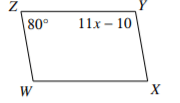10

300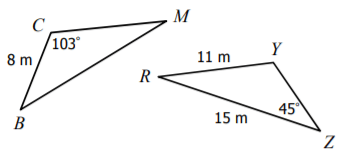If

∆BCM ≅ ∆ZYR, CM=?

11 m

300

Reasons 4 and 5Definition of midpoint and SSS

300

Reason 4Substitution property

300

Reasons 4 and 54. Substitution Property or Transitive Property

5. Definition of Complementary

300

Find the length of WZ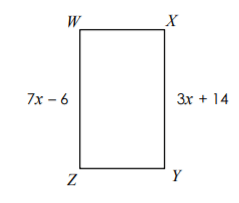29

400Given ∆BCM ≅ ∆ZYR,

m\angleB=?

45^\circ?

400

Reasons 1, 2, and 3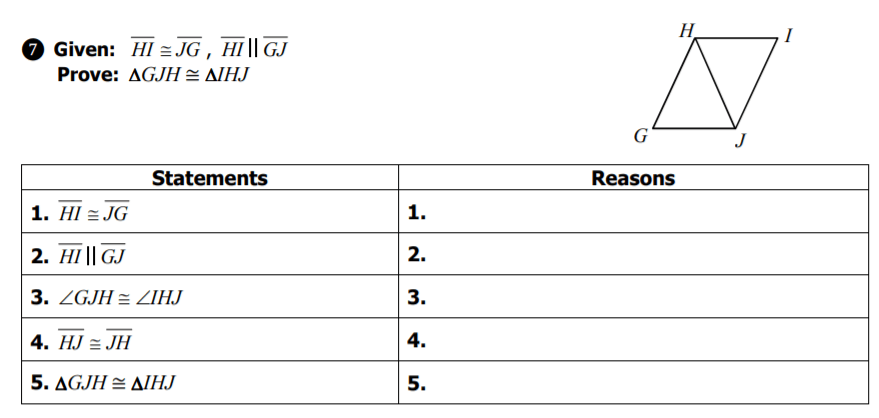Given, Given, and Alternate Interior Angles Theorem

400

Reasons 1 and 2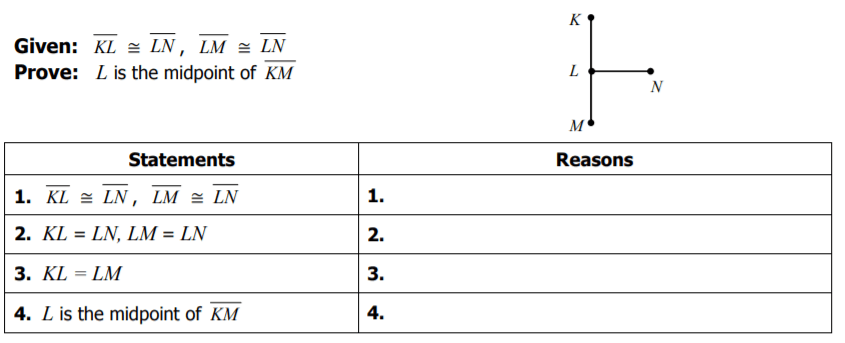Given and definition of congruence

400

Reasons 1 and 3 and Reasons 2 and 4?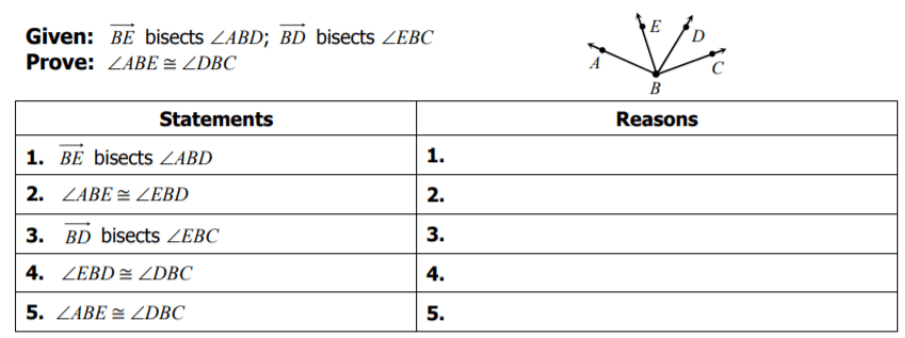1 and 3.) Given

2 and 4.) Definition of angle bisector

400

Find x.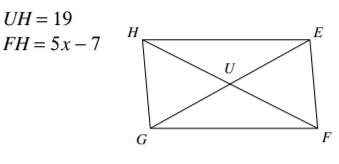9

500

Given ∆XPS ≅ ∆DNF, find the values of x and y.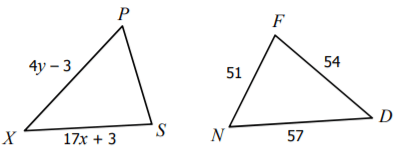x=3

y=15

500

Reasons 4 and 5Reflexive property and SAS

500

Reasons 3 and 4Transitive property and definition of midpoint

500

Theorem that justifies this statement:Congruent complements theorem

500

Find m<NML22

Click to zoom